# Spectrum of an operator

(diff) ← Older revision | Latest revision (diff) | Newer revision → (diff)The set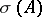of complex numbersfor which the operator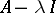does not have an everywhere-defined bounded inverse. Here,is a linear operator on a complex Banach spaceandis the identity operator on. Ifis not closed on, then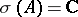, and therefore one usually considers spectra of closed operators (the spectrum of the closure of an operator for operators admitting a closure is sometimes called the closure spectrum).

Ifis either non-injective or non-surjective, then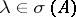. In the first caseis called an eigenvalue of; the set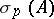of eigenvalues is called the point spectrum. In the second caseis called a point of the continuous spectrum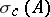or the residual spectrum, depending on whether the subspaceis dense inor not.

There are also other classifications of the points of a spectrum. For example,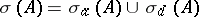, where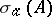consists of approximate eigenvalues (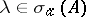if there are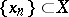with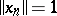such that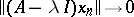), and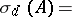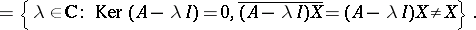Note that, and so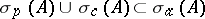. In perturbation theory, use is made of the limit spectrum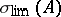, which consists of the limit points ofand the isolated eigenvalues of infinite multiplicity, of the Weyl spectrum, which is equal to the intersection of the spectra of all compact perturbations, etc.

Ifis a bounded operator, thenis compact and non-empty (in this casecoincides with the spectrum of the elementof the Banach algebra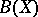, cf. Spectrum of an element); in general one can only say thatis closed in. On the set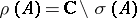one can define the analytic-valued function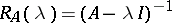, called the resolvent of(is called the resolvent set). With the help of resolvents a functional calculus foris built on functions analytic in a neighbourhood of: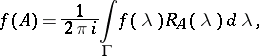whereis a contour enclosing(the unboundedness ofimposes restrictions on the choice of). Further conditions on the geometry of the spectrum and on the asymptotics of the resolvent enables one to extend this calculus.

The spectra of operator functions are defined by the formula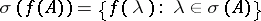(the spectral mapping theorem). The spectrumof the adjoint operator coincides withwhenis bounded; in general,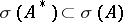.

If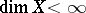, then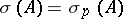, anddecomposes into the direct sum of subspaces invariant under, on each of whichinduces an operator with one-point spectrum. Spectral theory of operators is concerned with finding infinite-dimensional analogues for this decomposition. See also Spectral analysis; Spectral synthesis; Spectral operator; Spectral decomposition of a linear operator.

How to Cite This Entry:
Spectrum of an operator. Encyclopedia of Mathematics. URL: http://encyclopediaofmath.org/index.php?title=Spectrum_of_an_operator&oldid=11393
This article was adapted from an original article by V.S. Shul'man (originator), which appeared in Encyclopedia of Mathematics - ISBN 1402006098. See original article